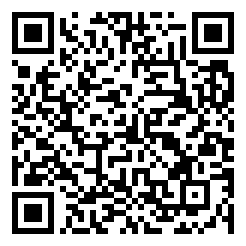Python入门2KeybrL 10月 08, 2017
• 在其它设备中阅读本文章
•SS::STA 2017第二次活动前半部分，关于Python入门

## 安全须知一

1. Python语句以换行结束，不需要也不能毫无缘由地在一个语句后面加上 ; 。当然，凡事总有例外，如果你需要在一行中书写多个语句，那么你需要用分号间隔他们。（但是原则上，如无特殊需求，尽量使用一行书写一个语句。关于一行多句以及一句多行的问题我们往后再谈。）
2. Python以代码的 缩进 确定层次结构（所以我们避免了 神圣的大括号之战 ），原则上同一缩进即为同一层（但我们仍然建议一个缩进为4个空格）。（所以你终于没有什么借口不缩进了吧）
3. Python 严格区分大小写 ，包括变量名、操作符、值、函数或类名、……（所有东西），比如 Truetrue 就完全不同（但我们仍然不希望你为 true 赋值）

## 玩耍前的热身

### 一点有用的内建函数

#### 输入输出

• print()
• input()

name = input('Your name:')
print('Hello %s!' % name)

Python2.7中 print 更像操作符，他可以不需要括号。而功能与 input() 类似的则是 raw_input()

#### 获取帮助

• help()

a = 1
help(a)

# 等价于下面这个
help(int)

# 可以help的东西很多
help(abs)
help(hhh)  # 这是什么鬼
help(help)
help(str)
help(list.append)

• exit()

### 关于代码注释

# 这是一个无聊的注释，他可以独立成行
print('Hello World!')  # 他也可以跟在语句后面
# 多行注释的每一行
# 都必须要有一个井号
# 就像这样
# 其实你也可以理解为，Python没有多行注释

• 独立成行的注释与语句一同缩进且在井号与注释内容之间留一空格
• 在语句后面的注释，在井号与前面的语句之间和与注释内容之间分别留两个和一个空格

### 初谈变量与常量

Python有一个官方库 keyword ，里面有一个保留字列表 kwlist 和一个判断是否为保留字的方法 iskeyword

import keyword
print(keyword.kwlist)  # 打印保留字列表
print(keyword.iskeyword('True'))  # 判断'True'是否为保留字

#### 灵活的变量

a = 233  # 整数
b = 1.33  # 浮点数
c = '233'  # 字符串

a = 233  # a是一个整数
print(a, type(a))
# 233 <class 'int'>

a = '哈哈哈'  # a变为一个字符串
print(a, type(a))
# 哈哈哈 <class 'str'>

a = 2.33  # a又变为一个浮点数
print(a, type(a))
# 2.33 <class 'float'>

#### 常量无用论

Python里没有常量，而唯一可以阻止一个变量被重新赋值的方法就是：你自己确保他没被重新赋值

PI = 3.14159265358979323846264338327950288419716939937510

### 标准类型

• int、float、bool、str（整型、浮点型、布尔型、字符串）
• list、tuple（列表、元组）
• dict（字典）
• NoneType（啥也不是）

#### 数字

int、float

Python中整数没有太多的禁忌（精度和大小方面），但是浮点数可能比较奇怪

a = 0x1234567890ABCDE
print(a)  # 可以使用16进制表示
b = 233323332333233323332333233323332333233323332333233323332333
print(b)  # 整数在精度和大小方面都没有限制
c = 2 ** 10000
print(c)  # 运算也无近似误差

d = 2.33333333333333333333333333333333333333333333  # 浮点数的精度会受限制
print(d)
e = 2.33E100
f = 2.33E500  # 指数一般不超过308（其实一般只能用到307）
print(e)
print(f)
# 运算起来也很神奇
print(2. ** 10000)
print(0.1 + 0.2)

print(2333333333333333333333333333333333333333333333333333333333333)
print(2333333333333333333333333333333333333333333333333333333333333.)

#### 字符串

Python中有现成的字符串类型（而且比C++的好用），你不用像C那样用字符数组、字符指针来操作字符串，你只需要

a = 'Hello World！'
print(a)

Python的字符串是一段在一对单引号或双引号之间的内容

a = '233哈哈哈'
b = "233哈哈哈"
print(a == b)  # 结果当然是True，他们是一样的

print('毋庸置疑的是: "在单引号标记的字符串里使用双引号是没有问题的"，其实反过来也可以')
print('但如果你既需要用双引号"，又要用单引号\'，那你将需要用反斜杠转义')

a = '这是第一行\n第二行\n还有第三行\n最后，第四行'
b = '''这是第一行

print(a)
print(b)

print('\\\t\\\\')
print(r'\\\t\\\\')

r 作为字符串的前缀可以使字符串内的反斜杠不转义

a = 'Hello %s!' % 'World'
print(a)

b = '%d %5d %010d' % (233, 233, 233)
print(b)

c = '%f %5.2f' % (2.33, 2.33)
print(c)

d = '%x' % 563
print(d)

a = 'abcdefghijklmn'
print(a[2:5:1])  # striing[start:end:step]
print(a[:6])  # 当start为0，end为字符串长度，step为1时他们可省略，省略step时其前的冒号亦可省略

# 用负数做出更复杂的切片
print(a[::-1])
print(a[-6:-2])

a = 'abcdefghijklmn'
print(a)
print(a[-3])

‘加’、‘数乘’

print('233' + '有趣吗')
print('233很无聊' * 2)

#### 列表和元组

a = [1, 2, 3, 4]
# 列表是由一组包裹在方括号内的数据组成的，他们不一定要有相同的类型
b = [1, '233', True, 2.345]
# 列表可以嵌套，类似于C的‘二维数组’
c = [[1, 2, 3], [2, 3, 4], [3, 4, 5]]

print(a)
print(c)
print(b[1:3])

“如果他真的那么智能，那他为什么不为我动态分配内存呢，他只需要检查我要加入的或者移出的数据的大小然后为我多申请一些内存或者释放多余的内存就可以了”

a.append(5)  # 在a的末尾加入5这个元素
a +=   # 作用类似于上面的方法，但这种方法是合并列表，而非追加元素
a.insert(2, 2.5)  # 将2.5插入到a的第2位，其后元素后移
a.pop(3)  # 删除a中第3位的数据，其后元素前移
a.update(1, 3)
# 还有一堆操作不讲了，自己help()和Google去

a = [2, 4, 1, 3, 5, 2, 6]
a.sort()
print(a)

a = (1, 2, 3, 4)
b = (1, '2', 3.0, 4, (1, 2, 3))
c = (233,)
print(a)
print(b[3:5])  # 切片、检索都是和列表完全一样的
print(c)  #  当元组中只有一个元素，唯一的元素后面必须写一个逗号，以消除歧义

a = ([1, 2, 3],)
a.append()
a.append()
print(a)

#### 字典

char keys;
int values;

a_dict = {
'name': '罗阳豪',
'email': 'keyboard-l@outlook.com',
}
print(a_dict['email'])

# 解释器会报错
print(a_dict['hhh'])

# 安全做法，使用get()方法查找不确定的键
print(a_dict.get('hhh'))

# 随意增改
a_dict['hhh'] = '233'

# 删除也很容易
a_dict.pop('hhh')
print(a_dict.get('hhh'))

### 标准类型操作符

a < b a小于b
a > b a大于b
a <= b a小于等于b
a >= b a大于等于b
a == b a等于b
a != b a不等于b
a is b a和b是同一个对象
a is not b a和b不是同一个对象

Python2.7还能使用<>表示‘不等于’，虽然我们并不推荐这么做

not a a的逻辑
a and b a和b的逻辑
a or b a和b的逻辑

a + b a加b的和（加法）
a - b a减b的差（减法）
a * b a乘b的积（乘法）
a / b a除以b的商（浮点除法）
a ** b a的b次方（乘方）
a // b a除以b的商（地板除法）
a % b a除以b的余（取模）

Python2.7中 / 表现地类似于C的除法（整数相除则地板除、浮点数相除或浮点数与整数相除则为浮点除法）

### 再谈变量

Python的变量与C语言的变量看起来有点类似（但几乎完全不同）

#### 强类型、动态类型

• Python不需要显式的变量类型声明（隐式）
• 变量的类型在赋值时确定，且可以通过赋值随意改变一个变量的类型（动态）
• 每个变量都拥有明确的类型且不允许隐式的类型转换（强）

（老实说，我偶尔会看的...）